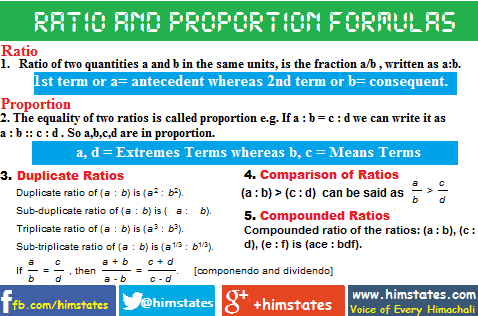Himstates website is aspired to provide you competitive exams information, questions and answers ,formulas etc. Ratio and proportion is an important topic for competitive exams, entrance exams as well interviews.
So to benefit students formulas , questions and previous year questions on the topic of 'Ratio and Proportion' is given below,
• [message]
• 'Ratio and proportion' important in which Competitive Exams ?
• Bank Competitive Exam
UPSC Competitive Exams
SSC Competitive Exams
Defense Competitive Exams
LIC or GIC  Exams
University Grants Commission or UGC
Common Aptitude Test or CAT
Career Aptitude Test
Railway Competitive Exam
MAT, GMAT, IBPS Exam, CSAT, CLAT

Ratio and proportion aptitude questions are also important in state(Himachal Pradesh) competitive exams of HPPSC, HPSSSB, HPBoSE and many more exams of Banking as well as entrance exams etc.

###Fourth Proportional : If a : b = c : d, then d is called the fourth proportional to a, b, c.
Third Proportional : a : b = c : d, then c is called the third proportion to a and b.
Mean Proportional: Mean proportional between a and b is ab.

### Questions On Topic ' Ratio and Proportion'

Q. 1.  a and b are two numbers , respectively 20% and 50% more than third number. The ratio of two numbers a and b is
A. 3 : 5
B. 4 : 5  (Ans)
C. 6 : 5
D. 6 : 7

Q. 2. Sunil and Anil have total amonut Rupees 1210 , If 4/15 of Sunil's amount is equal to 2/5 of Anil's amount. How much amount of money Anil have
A. Rs. 470
B. Rs. 575
C. Rs. 484  (Ans)
D. Rs. 525

Q. 3. Sum of money is distributed among Rohit, Ritika, Vibhuti and Shrawan  in proportion of 5 : 2 : 4 : 3 , If Vibhuti gets Rupees 1000 more than Shrawan , what is share of Ritika ?
A. Rs. 1500
B. Rs. 500
C. Rs. 1000
D. Rs. 2000  (Ans)

Q. 4. In a government college seats for Arts , Commerce and Science are in ratio of 5 : 7 : 8 . There is proposal of seats increase by college management by 40%, 50% and 75% respectively. Find ratio of increased seats in college
A. 2 : 5 : 7
B. 5 : 7 : 8
C. 2 : 3 : 4  (Ans)
D. 6 : 7 : 8

Q. 5. If 6 : x  :: 2 : 3 than find value of  x
A.  7
B.  8
C.  6
D.  9  (Ans)

Q. 6. Sum of a, b and c numbers is 98.If a : b = 2:3 and b : c = 5 : 8 , than b is
A. 20
B. 30  (Ans)
C. 35
D. 45

Q. 7. If amount 782 is divided in three parts , proportional to 1/2 : 2/3 : 3/4 than first part is
A. Rs. 182
B. Rs. 196
C. Rs. 192
D. Rs. 204  (Ans)

Q. 8. Find fourth proportional to 5, 10, 15
A. 15
B. 20
C. 25
D. 30  (Ans)

Q. 9. In a box there are coins of 25 paisa , 10 paisa and 5 paisa in the ratio of 1 : 2: 3 . If there is 30 Rs. in all , Find how many 5 paisa are there
A. 75  (Ans)
B. 100
C. 125
D. 150

Q. 10. If 40 % of a number is equal to 2/3 of another number , than find out ratio of first number to second number.
A. 2 : 3
B. 5 : 7
C. 5 : 3  (Ans)
D. 2 : 5
[next]
Q. 11. If a:b = 2:7 and b:c = 6:7 than find a:b:c ?
A. 12 : 42 :49  (Ans)
B. 14 : 49 :33
C. 12 : 49 :50
D. None of these

Q. 12. The Duplicate Ratio of 4 : 7 ?
A. 4: 7
B. 2 : 7/2
C. 16 : 49  (Ans)
D. None of these

Q. 13. The Triplicate Ratio of 2 : 3 ?
A. 4: 9
B. 8 : 27  (Ans)
C. 3 : 2
D. All of the above

Q. 14. The Sub - Duplicate Ratio of  4 : 25 ?
A. 2 : 5  (Ans)
B. 16 : 125
C. 64 : 625
D. All of the above

Q. 15. Third Proportional to 1 and 2 is ?
A. 2
B. 3
C. 4  (Ans)
D. All of the above
Q. 16. Fourth Proportional to 2, 4 and 8 is ?
A. 12
B. 16
C. 10
D. None of these